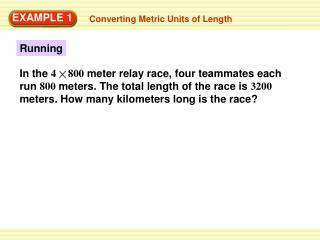DownloadDownload PresentationRunning

# Running

Télécharger la présentation## Running

- - - - - - - - - - - - - - - - - - - - - - - - - - - E N D - - - - - - - - - - - - - - - - - - - - - - - - - - -
##### Presentation Transcript

1. In the 4800 meter relay race, four teammates each run 800 meters. The total length of the race is 3200 meters. How many kilometers long is the race? EXAMPLE 1 Converting Metric Units of Length Running

2. The 4 800 meter relay race is 3.2 kilometers long. From meters to kilometers, the decimal point is moved 3 placesto the left, so divide by 103, or 1000. 3200 1000 = 3.2 EXAMPLE 1 Converting Metric Units of Length To find the length in kilometers of the relay race, convert 3200 meters to kilometers. SOLUTION You are converting from a smaller unit (meters) to a larger unit (kilometers), so divide by a power of 10. 3200 meters = 3.2 kilometers ANSWER

3. a. 15 g = ? mg b. 590 mL = ? L 15 1000 = 15,000, so 15 g = 15,000 mg. 590 1000 = 0.59, so 590 mL = 0.59 L. EXAMPLE 2 Converting Units of Mass and Capacity Copy and complete the statement. SOLUTION a. To convert from grams to milligrams, multiply by1000. b. To convert from milliliters to liters, divide by 1000.

4. 1. 6800 m = ? km 2. 54 m = ? mm 3. 830 cm = ? m ANSWER ANSWER ANSWER 6.8 54,000 8.3 GUIDED PRACTICE for Examples 1 and 2 Copy and complete the statement.

5. ANSWER ANSWER ANSWER 11.5 0.1 9250 4. 115 mm = ? cm 5. 9.25 kL = ? L 6. 100 g = ? kg GUIDED PRACTICE for Examples 1 and 2 Copy and complete the statement.